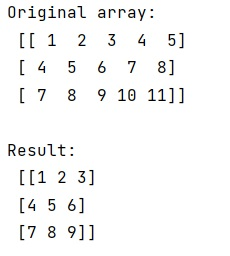# Extracting first n columns of a NumPy matrix

Learn how to extract first n columns of a NumPy matrix in Python?
Submitted by Pranit Sharma, on February 09, 2023

NumPy is an abbreviated form of Numerical Python. It is used for different types of scientific operations in python. Numpy is a vast library in python which is used for almost every kind of scientific or mathematical operation. It is itself an array which is a collection of various methods and functions for processing the arrays.

## NumPy Matrix - Extracting first n columns

Suppose that we are given a multi-dimensional numpy array with certain rows and columns and we just need to extract the first n columns of this numpy array.

Slicing is the technique that will help us to find the first n columns of this numpy array.

Suppose that we have an array a which contains 5 columns and we need to extract its first 3 columns, we will use the following slicing code to extract the first 3 columns: a[: , : , 3]

Let us understand with the help of an example,

## Python code to extract first n columns of a NumPy matrix

```# Import numpy
import numpy as np

# Creating a numpy array
arr =  np.array([[1, 2, 3, 4, 5],
[4, 5, 6, 7, 8],
[7, 8, 9, 10, 11]])

# Display original array
print("Original array:\n",arr,"\n")

# Slicing the array and extracting
# first 3 columns
res = arr[:,:3]

# Display Result
print("Result:\n",res,"\n")
```

Output: